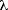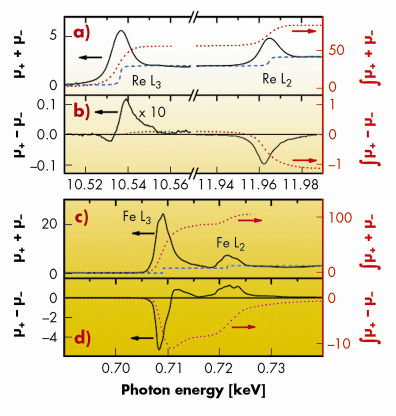Double perovskites are materials of the form A2BB’O6, where the transition metal atoms B and B’ alternate between neighbouring oxygen octahedra, as depicted in Figure 114. Some double perovskites have their conduction band fully spin polarised . The Re-based double perovskites in particular have attracted attention due to their fairly high transition temperature from a paramagnetic to ferrimagnetic state (513 K for Ca2FeReO6), which makes this family of materials candidates for future spintronics applications. We studied the compound Ba2FeReO6 using X-ray magnetic circular dichroism (XMCD) at the L2,3 edges of both Re and Fe, associated with synchrotron and neutron X-ray powder diffraction. The neutron diffraction was performed on the BT-1 diffractometer at the NIST Center for Neutron Research and the X-ray diffraction data was taken at the XPD beamline of the Laboratório Nacional de Luz Síncrontron (LNLS). The XMCD data at Re and Fe L2,3 edges was measured at the DXAS beamline at LNLS and beamline ID08 at the ESRF, respectively.Fig. 114: Crystalline structure of a double perovskite A2BB’O6. The transition metal atoms B and B’ are in the centre of alternating oxygen octahedras. The cation A occupies the interstices between the octahedras.

A clear transition from the cubic to a tetragonal structure was evident in the diffraction data when we decreased the temperature below 309 K, which is also the paramagnetic to ferrimagnetic transition temperature. Magnetic field dependent X-ray powder diffraction proved that both transitions are related, and also that a quite strong magnetoelastic effect develops below the transition temperature. Also from these diffraction measurements, we obtained a linear magnetoelastic constant100 = 1.1 x 103, which is a giant value for transition metal based metallic systems.

Usually magnetoelastic effects are associated with strong spin-lattice interactions, which take place through the spin-orbit coupling. Therefore, we anticipated the presence of non-negligible orbital moments in the system. To investigate such spin-lattice interactions we measured the XMCD at both Re and Fe L2,3 edges. XMCD allowed us to discriminate the contribution of each element in the material to the total magnetisation. By applying well-established sum rules, we evaluated separately the spin and orbital contribution of Fe and Re.

Figure 115 shows the isotropic X-ray absorption spectra of Re and Fe measured at 10 K and the corresponding XMCD signals, which are shown as continuous lines. The integrals used to calculate the sum rules are shown as dotted red lines in these figures. It is very interesting to note that the orbital moment of the probed atom is directly proportional to the integral of the XMCD signal. From the red dotted lines in Figures 115b and 115d one can see that this integral has a non negligible value in the case of Re, whereas it is almost zero for Fe. This direct result let us conclude that the Re orbital moment is responsible for the unusual magnetoelastic behaviour in this sample. To obtain quantitative results for the spin and orbital moments we employed sum rules based on the integral values shown in Figure 115. For Fe we found spin and orbital moments of mspin = 2.8(2) µB and morb = 0.04(2) µB. In the case of Re, mspin = -0.64(4) µB and morb = 0.19(1) µB. The negative value for the spin moment of Re simply reflects the ferrimagnetic alignment between Fe and Re atoms in the sample. It is also worth noting the size of the Re orbital moment. Indeed, the ratio morb/mspin is -0.293 for Re and 0.013 in the case of Fe. Thus the orbital moment is quite large for Re, whereas it is almost quenched for Fe. One must remember that the 5d transition metals are on the verge of becoming magnetic. Nonetheless, even in the case of Re impurities in Fe, the morb/mspin ratio is calculated to be about ten times smaller than what was found in our measurements .Fig. 115: X-ray absorption and X-ray magnetic circular dichroism at Re (top) and Fe (bottom). The red dotted lines indicate the integrals used in the sum rules calculations.

Based on the information obtained from XMCD and diffraction we can draw two important conclusions: i) calculations show that the conduction band in Ba2FeReO6 has an Re 5d character, which means that in this material the conduction electrons carry an appreciable orbital momentum; ii) in analogy to the manganites, which also present a huge magnetoelestic effect, we can suggest that Ba2FeReO6 is on the verge of developing an orbital ordering state.

References

 K.-I. Kobayashi, T. Kimura, H. Sawada, K. Terakura and Y. Tokura, Nature 395, 677 (1998).
 H. Ebert, R. Zeller, B. Drittler and P.H. Dederichs, J. Phys. 67, 4576 (1990).

Principal publication and authors

C. Azimonte (a,b), J.C. Cezar (c), E. Granado (a,b), Q. Huang (d), J.W. Lynn (d,e), J.C.P. Campoy (a), J. Gopalakrishnan (e,f), and K. Ramesha (f), Phys. Rev. Lett. 98, 017204 (2007).
(a) Instituto de Física “Gleb Wataghin”, UNICAMP, Campinas (Brazil)
(b) Laboratório Nacional de Luz Síncrotron, Campinas (Brazil)
(c) ESRF
(d) NIST Center for Neutron Research, Gaithersburg (USA)
(e) Center for Superconductivity Research, University of Maryland (USA)
(f) Solid State and Structural Chemistry Unit, Indian Institute of Science, Bangalore (India)